# 使用机器学习来预测股票价格

2018/11/28 11:11#### 工作流程

1.获取股票价格数据；

2.使用小波变换去噪数据；

3.使用Stacked Autoencoders提取特征；

4.使用特征训练LSTM；

5.测试预测准确性的模型；

#### 1.数据采集

pandas_datareader是借助雅虎财经的API，它可以很容易获得股票价格数据，只需使用以下命令即可完成：

stock_data = pdr.get_data_yahoo(self.ticker, self.start, self.end)

#### 小波变换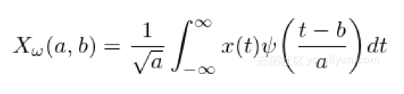1.使用小波变换来变换数据；

2.消除超过完全标准偏差的系数（在所有系数中）；

3.反变换新系数以获得去噪数据；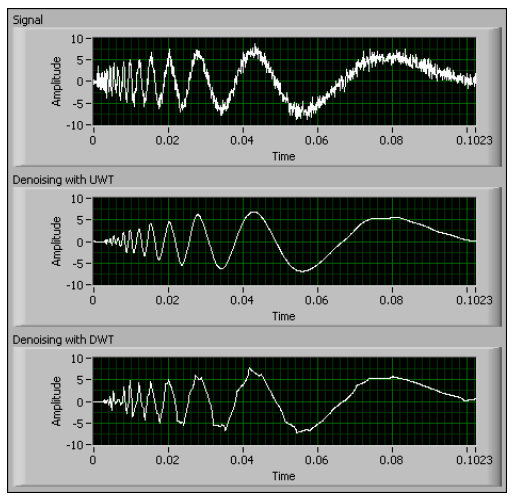x = np.array(self.stock_data.iloc[i: i + 11, j]) (ca, cd) = pywt.dwt(x, "haar") cat = pywt.threshold(ca, np.std(ca), mode="soft") cdt = pywt.threshold(cd, np.std(cd), mode="soft") tx = pywt.idwt(cat, cdt, "haar")

#### 堆叠式自动编码器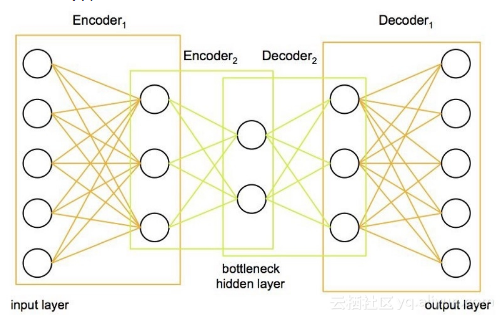class AutoEncoder: def __init__(self, encoding_dim): self.encoding_dim = encoding_dim def build_train_model(self, input_shape, encoded1_shape, encoded2_shape, decoded1_shape, decoded2_shape): input_data = Input(shape=(1, input_shape)) encoded1 = Dense(encoded1_shape, activation="relu", activity_regularizer=regularizers.l2(0))(input_data) encoded2 = Dense(encoded2_shape, activation="relu", activity_regularizer=regularizers.l2(0))(encoded1) encoded3 = Dense(self.encoding_dim, activation="relu", activity_regularizer=regularizers.l2(0))(encoded2) decoded1 = Dense(decoded1_shape, activation="relu", activity_regularizer=regularizers.l2(0))(encoded3) decoded2 = Dense(decoded2_shape, activation="relu", activity_regularizer=regularizers.l2(0))(decoded1) decoded = Dense(input_shape, activation="sigmoid", activity_regularizer=regularizers.l2(0))(decoded2) autoencoder = Model(inputs=input_data, outputs=decoded) encoder = Model(input_data, encoded3) # Now train the model using data we already preprocessed autoencoder.compile(loss="mean_squared_error", optimizer="adam") train = pd.read_csv("preprocessing/rbm_train.csv", index_col=0) ntrain = np.array(train) train_data = np.reshape(ntrain, (len(ntrain), 1, input_shape)) # print(train_data) # autoencoder.summary() autoencoder.fit(train_data, train_data, epochs=1000)

#### 4. LSTM模型

LSTM模型不需要介绍，因为它在预测时间序列中变得非常普遍和流行。它从细胞状态的存在中获得了卓越的预测能力，使其能够理解和学习数据的长期趋势。这对我们的股票价格数据尤为重要,下面我将讨论我认为重要的设计选择的某些方面。

#### 优化器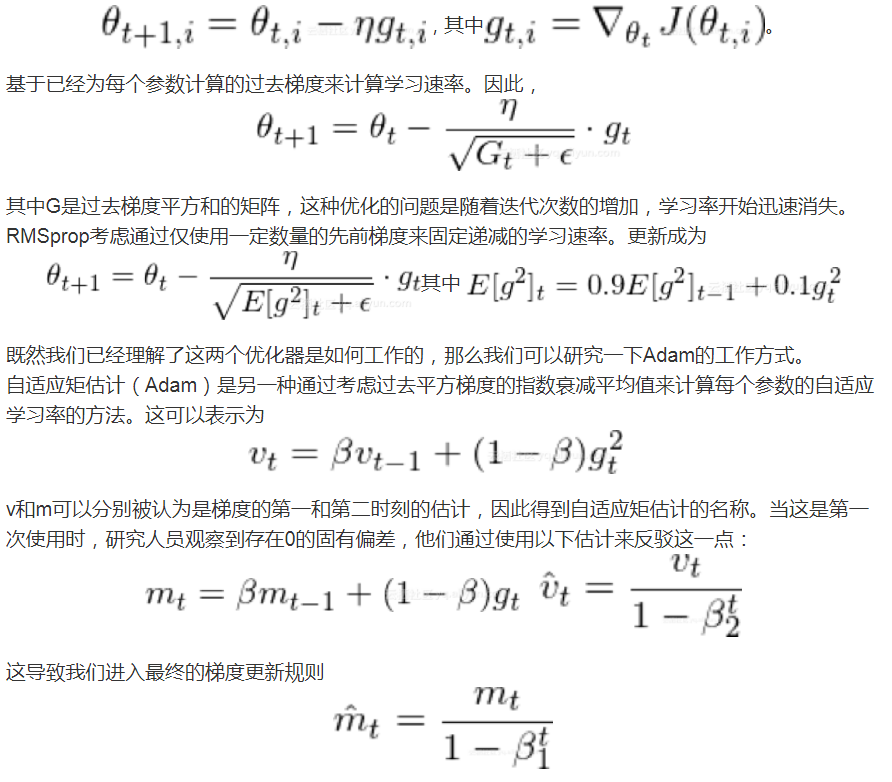1.Â  每个参数和每次迭代的学习率都不同；

3.Â  梯度更新使用权重分布矩阵。

#### 正则化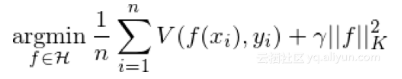#### Dropout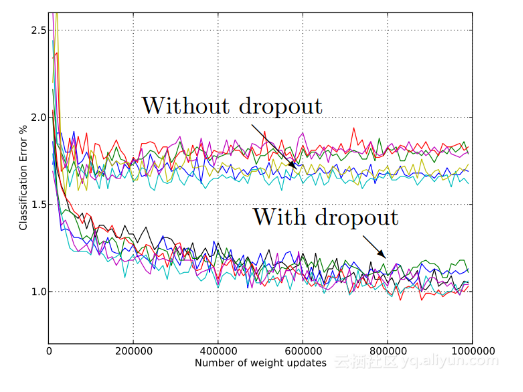#### 5.模型实施

class NeuralNetwork: def __init__(self, input_shape, stock_or_return): self.input_shape = input_shape self.stock_or_return = stock_or_return def make_train_model(self): input_data = kl.Input(shape=(1, self.input_shape)) lstm = kl.LSTM(5, input_shape=(1, self.input_shape), return_sequences=True, activity_regularizer=regularizers.l2(0.003), recurrent_regularizer=regularizers.l2(0), dropout=0.2, recurrent_dropout=0.2)(input_data) perc = kl.Dense(5, activation="sigmoid", activity_regularizer=regularizers.l2(0.005))(lstm) lstm2 = kl.LSTM(2, activity_regularizer=regularizers.l2(0.01), recurrent_regularizer=regularizers.l2(0.001), dropout=0.2, recurrent_dropout=0.2)(perc) out = kl.Dense(1, activation="sigmoid", activity_regularizer=regularizers.l2(0.001))(lstm2) model = Model(input_data, out) model.compile(optimizer="adam", loss="mean_squared_error", metrics=["mse"]) # load data train = np.reshape(np.array(pd.read_csv("features/autoencoded_train_data.csv", index_col=0)), (len(np.array(pd.read_csv("features/autoencoded_train_data.csv"))), 1, self.input_shape)) train_y = np.array(pd.read_csv("features/autoencoded_train_y.csv", index_col=0)) # train_stock = np.array(pd.read_csv("train_stock.csv")) # train model model.fit(train, train_y, epochs=2000)

#### 结果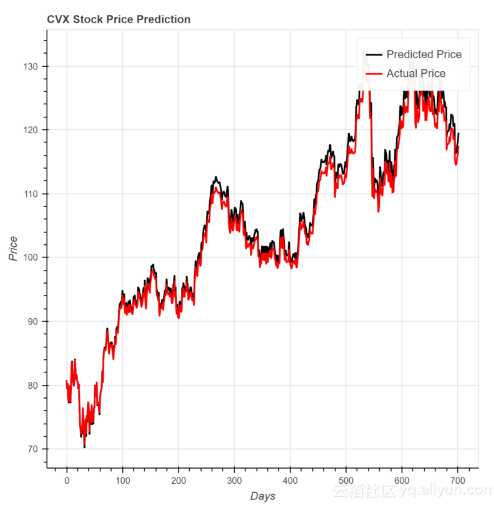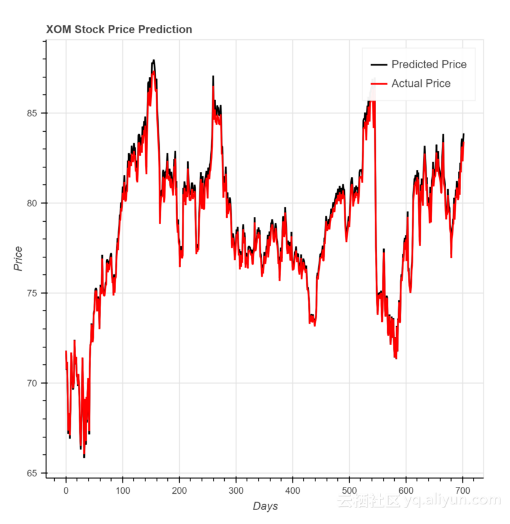0
0 收藏

### 作者的其它热门文章0 评论
0 收藏
0